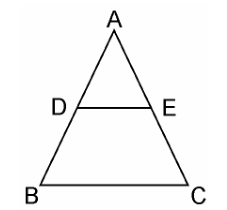Guru

# Using theorem 6.1, prove that a line drawn through the mid-points of one side of a triangle parallel to another side bisects the third side. (Recall that you have proved it in Class IX). Q.7

• 0

Using theorem how i solve the problem of exercise 6.2 of triangles chapter, how i solve this problem because it is very important Using Basic proportionality theorem, prove that a line drawn through the mid-points of one side of a triangle parallel to another side bisects the third side. (Recall that you have proved it in Class IX).

Share

1.Solution:

Given, in ΔABC, D is the midpoint of AB such that AD=DB.

A line parallel to BC intersects AC at E as shown in above figure such that DE || BC.

We have to prove that E is the mid point of AC.

Since, D is the mid-point of AB.

⇒AD/DB = 1 …………………………. (i)

In ΔABC, DE || BC,

By using Basic Proportionality Theorem,

Therefore, AD/DB = AE/EC

From equation (i), we can write,

⇒ 1 = AE/EC

∴ AE = EC

Hence, proved, E is the midpoint of AC.

• 0